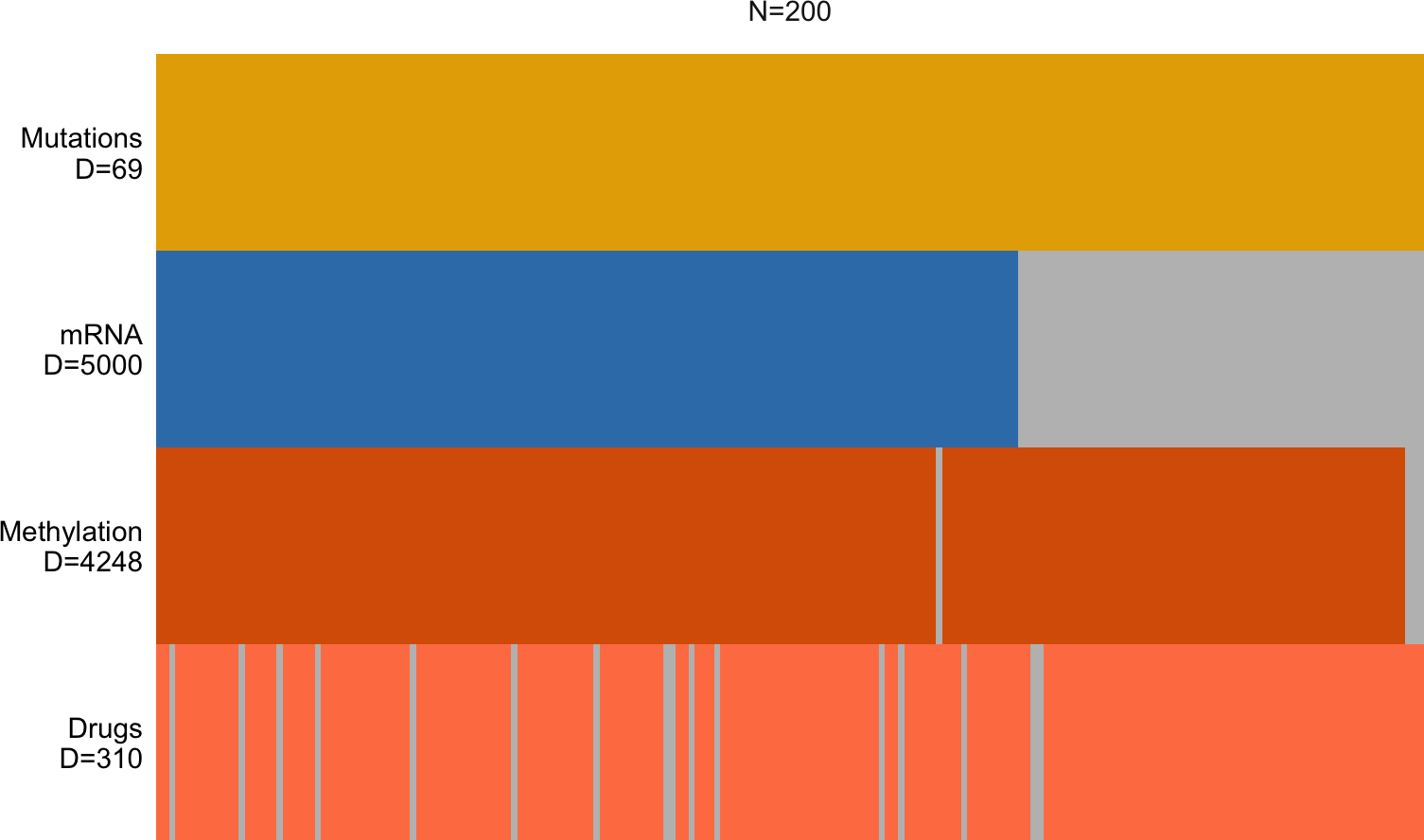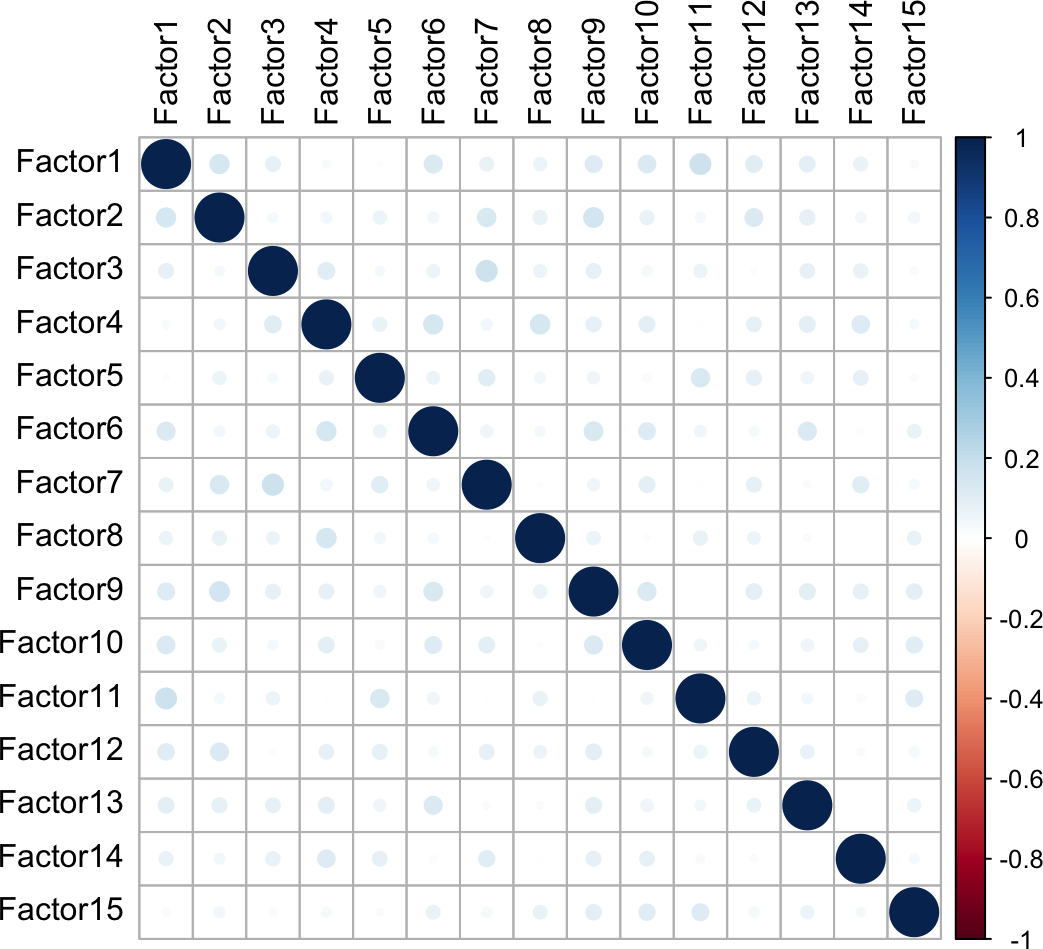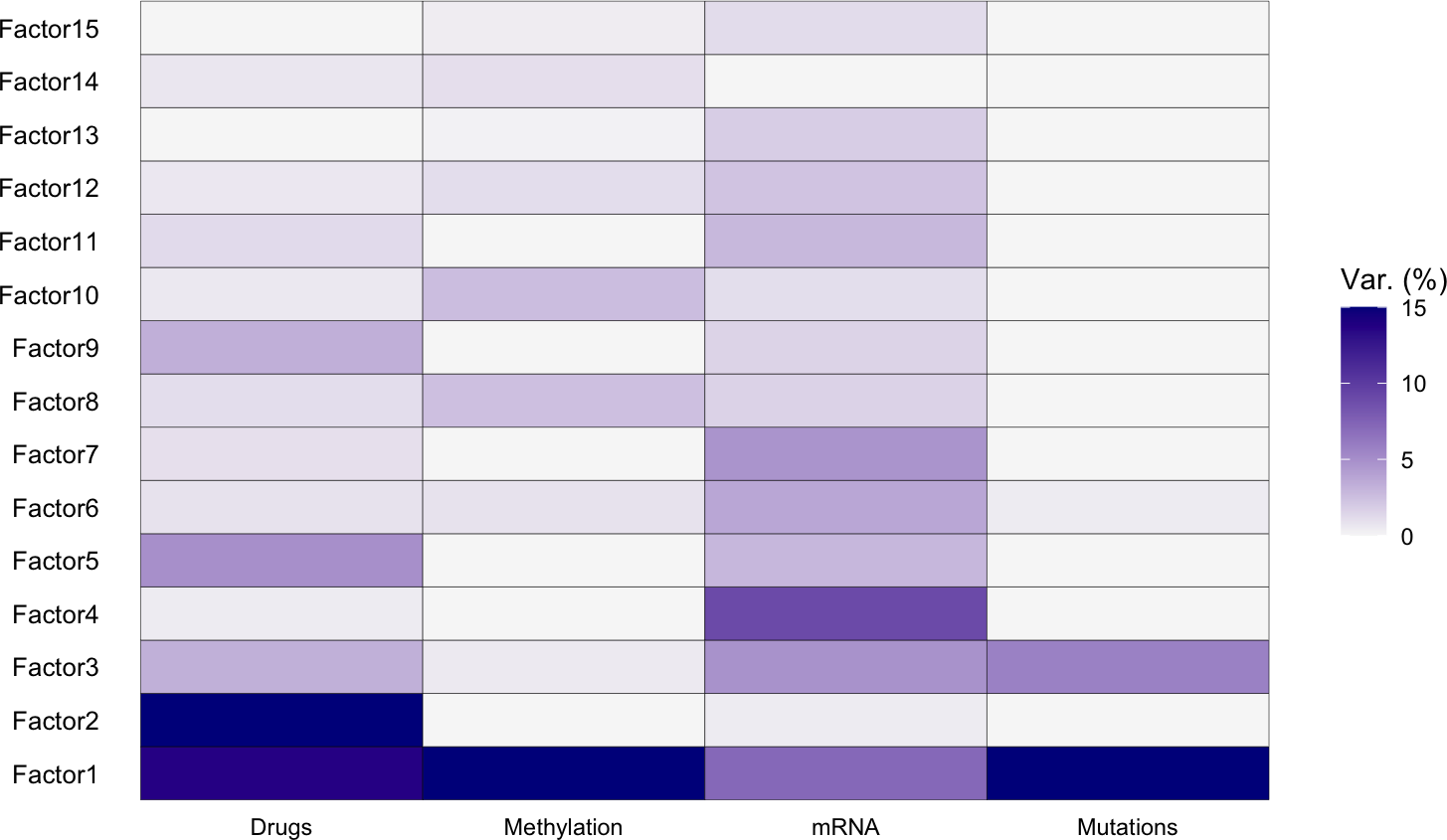# 1 Introduction

This vignette show how to use MOFA+ on the bulk multi-omics data set that was used in the first publication of MOFA and the original vignette of the MOFA package.

Briefly, the data consists of four omics including DNA methylation, RNA-seq, somatic mutations and drug response data from blood for N=200 patients with Chronic Lymphocytic Leukemia (CLL). The data set is explained in detail in this article and is publicly available here

# 2 Load libraries and data

``````library(MOFA2)
library(data.table)
library(ggplot2)
library(tidyverse)``````

Data is stored as a list of matrices. Features are stored in the rows and samples in the columns

``````utils::data("CLL_data")
lapply(CLL_data,dim)``````
``````## \$Drugs
##  310 200
##
## \$Methylation
##  4248  200
##
## \$mRNA
##  5000  200
##
## \$Mutations
##   69 200``````

Sample metadata are stored as a data.frame. Important columns are:

• Gender: m (male), f (female)
• Age: age in years
• TTT: time (in years) which passed from taking the sample to the next treatment
• TTD: time (in years) which passed from taking the sample to patients’ death
• treatedAfter: (TRUE/FALSE)
• Died: whether the patient died (TRUE/FALSE)

The full meta data can be obtained from the Bioconductor package `BloodCancerMultiOmics2017` as `data("patmeta")`.

``CLL_metadata <- fread("ftp://ftp.ebi.ac.uk/pub/databases/mofa/cll_vignette/sample_metadata.txt")``

# 3 Create the MOFA obejct and train the model

Create the MOFA object

``MOFAobject <- create_mofa(CLL_data)``
``MOFAobject``
``````## Untrained MOFA model with the following characteristics:
##  Number of views: 4
##  Views names: Drugs Methylation mRNA Mutations
##  Number of features (per view): 310 4248 5000 69
##  Number of groups: 1
##  Groups names: group1
##  Number of samples (per group): 200``````

## 3.1 Plot data overview

Visualise the number of views (rows) and the number of groups (columns) exist, what are their corresponding dimensionalities and how many missing information they have (grey bars).

``plot_data_overview(MOFAobject)``## 3.2 Define MOFA options

### 3.2.1 Data options

Important arguments:

• scale_groups: scale groups to the same total variance? Default is `FALSE`
• scale_views: scale views to the same total variance? Default is `FALSE`
• views: views names
• groups: groups names
``````data_opts <- get_default_data_options(MOFAobject)
data_opts``````
``````## \$scale_views
##  FALSE
##
## \$scale_groups
##  FALSE
##
## \$views
##  "Drugs"       "Methylation" "mRNA"        "Mutations"
##
## \$groups
##  "group1"``````

### 3.2.2 Model options

Important arguments:

• num_factors: number of factors
• likelihoods: likelihood per view (options are “gaussian”, “poisson”, “bernoulli”). By default they are inferred automatically.
• spikeslab_factors: use spike-slab sparsity prior in the factors? default is `FALSE`.
• spikeslab_weights: use spike-slab sparsity prior in the weights? default is `TRUE`.
• ard_factors: use ARD prior in the factors? Default is `TRUE` if using multiple groups.
• ard_weights: use ARD prior in the weights? Default is `TRUE` if using multiple views.
``````model_opts <- get_default_model_options(MOFAobject)
model_opts\$num_factors <- 15

model_opts``````
``````## \$likelihoods
##       Drugs Methylation        mRNA   Mutations
##  "gaussian"  "gaussian"  "gaussian" "bernoulli"
##
## \$num_factors
##  15
##
## \$spikeslab_factors
##  FALSE
##
## \$spikeslab_weights
##  TRUE
##
## \$ard_factors
##  FALSE
##
## \$ard_weights
##  TRUE``````

### 3.2.3 Training options

Important arguments:

• maxiter: number of iterations. Default is 1000.
• convergence_mode: “fast”, “medium” (default), “slow”. For exploration, the fast mode is good enough.
• seed: random seed
``````train_opts <- get_default_training_options(MOFAobject)
train_opts\$convergence_mode <- "slow"
train_opts\$seed <- 42

train_opts``````
``````## \$maxiter
##  1000
##
## \$convergence_mode
##  "slow"
##
## \$drop_factor_threshold
##  -1
##
## \$verbose
##  FALSE
##
## \$startELBO
##  1
##
## \$freqELBO
##  1
##
## \$stochastic
##  FALSE
##
## \$gpu_mode
##  FALSE
##
## \$seed
##  42
##
## \$outfile
## NULL
##
## \$save_interrupted
##  FALSE``````

## 3.3 Train the MOFA model

Prepare the MOFA object

``````MOFAobject <- prepare_mofa(MOFAobject,
data_options = data_opts,
model_options = model_opts,
training_options = train_opts
)``````

Train the model: this should take ~2min

``````# NOTE: The software has evolved since the original publication and the results will not be 100% identical to the original publication, please use the pretrained model if you are running through the vignette for the fist time
# saveRDS(MOFAobject,"MOFA2_CLL.rds")

# 4 Overview of the trained MOFA model

## 4.1 Slots

The MOFA object consists of multiple slots where relevant data and information is stored. For descriptions, you can read the documentation using `?MOFA`. The most important slots are:

• data: input data used to train the model (features are centered at zero mean)
• expectations: expectations of the posterior distributions for the Weights and the Factors
``slotNames(MOFAobject)``
``````##   "data"               "intercepts"         "imputed_data"
##   "training_stats"     "training_options"   "stochastic_options"
##  "data_options"       "model_options"      "dimensions"
##  "on_disk"            "dim_red"            "cache"
##  "status"``````

Data:

``names(MOFAobject@data)``
``##  "Drugs"       "Methylation" "mRNA"        "Mutations"``
``dim(MOFAobject@data\$Drugs\$group1)``
``##  310 200``

Factor and Weight values (expectations of the posterior distributions):

``names(MOFAobject@expectations)``
``##  "Z" "W"``
``````# Dimensionality of the factor matrix: 200 samples, 15 factors
dim(MOFAobject@expectations\$Z\$group1)``````
``##  200  15``
``````# Dimensionality of the mRNA Weight matrix: 5000 features, 15 factors
dim(MOFAobject@expectations\$W\$mRNA)``````
``##  5000   15``

The sample metadata must be provided as a data.frame and it must contain a column `sample` with the sample IDs. Make sure that the samples in the metadata match the samples in the model

``````# Sanity check

## 4.3 Correlation between factors

A good sanity check is to verify that the Factors are largely uncorrelated. In MOFA there are no orthogonality constraints such as in Principal Component Analysis, but if there is a lot of correlation between Factors this suggests a poor model fit. Reasons? Perhaps you used too many factors or perhaps the normalisation is not adequate.

``plot_factor_cor(MOFAobject)``## 4.4 Plot variance decomposition

### 4.4.1 Variance decomposition by Factor

The most important insight that MOFA generates is the variance decomposition analysis. This plot shows the percentage of variance explained by each factor across each data modality (and group, if provided). It summarises the sources of variation from a complex heterogeneous data set in a single figure.

``plot_variance_explained(MOFAobject, max_r2=15)``What insights from the data can we learn just from inspecting this plot?

• Factor 1 captures a source of variability that is present across all data modalities. Thus, its etiology is likely to be something very important for the disease
• Factor 2 captures a very strong source of variation that is exclusive to the drug response data.
• Factor 3 captures variation that is present across multiple data modalities, except for DNA methylation. This is likely to be important too.
• Factor 5 is capturing some co-variation between the mRNA and the drug response assay.

### 4.4.2 Total variance explained per view

A reasonable question is whether the model is providing a good fit to the data. For this we can plot the total variance explained (using all factors). The resulting values will depend on the nature of the data set, the number of samples, the number of factors, etc. Some general guidelines:

• Noisy data sets with strong non-linearities will result in small amounts of variance explained (<10%).
• The higher the number of samples the smaller the total variance explained
• The higher the number of factors, the higher the total variance explained.
• MOFA is a linear and sparse model. This is helpful to prevent overfitting, but it will never explain 100% of the variance, even if using a lot of Factors.

In this data set, using only K=15 factors the model explains up to ~54% of the variation in the Drug response and ~42% of the variation in the mRNA data. This is quite remarkable for a linear model.

``plot_variance_explained(MOFAobject, plot_total = T)[]``